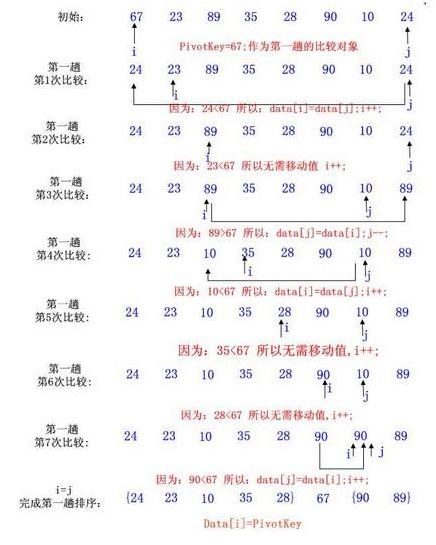# 排序算法之快速排序原理与实战

JAVA 3376浏览```package com.xttblog.sort;

public class QuickSort {
//快速排序
private static void quickSort(int s[], int l, int r){
if(l>=r)
return;
//将中间的这个数和第一个数交换 参见注1
int i = l, j = r, x = s[l];
while (i < j){
while(i < j && s[j] >= x) // 从右向左找第一个小于x的数
j--;
if(i < j)
s[i++] = s[j];

while(i < j && s[i] < x) // 从左向右找第一个大于等于x的数
i++;
if(i < j)
s[j--] = s[i];
}
s[i] = x;
quickSort(s, l, i - 1); // 递归调用
quickSort(s, i + 1, r);
}

public static void main(String[] args) {
int s [] = {67,23,89,35,28,90,10,24};
for (int i : s) {
System.out.print(i+" ");
}
quickSort(s,0,7);
System.out.println();
for (int i : s) {
System.out.print(i+" ");
}
//排序前后输入结果为：
//67 23 89 35 28 90 10 24
//10 23 24 28 35 67 89 90
}
}```